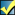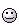A Flash Developer Resource Site

# Thread: [RESOLVED] Calling a function from a name in text box?

1. ##[RESOLVED] Calling a function from a name in text box?

I have a text box on the stage

i want to be able to type the name of a function and it's arguments, click a button, and have the function executed with said argruments
is this possible?

Something like this:An input box and a button, button is clicked and function "function1" is called with argruments "hello" and 1
alternatly i could have more than one text box, one for the function name, and others for the arguments, if that'd be easier

thanksReply With Quote

2. where you have function1("hello",1) in textfield instance - txt
PHP Code:
``` var a:String = txt.text.split("(").join(",") var b:String = a.split(")").join(""); var arr:Array = b.split(","); var c:String = arr; root[c](arr,arr); function function1(n,m){ trace(n+"  "+m); };  ```Reply With Quote

3.Originally Posted by a_modified_dogwhere you have function1("hello",1) in textfield instance - txt
PHP Code:
``` var a:String = txt.text.split("(").join(",") var b:String = a.split(")").join(""); var arr:Array = b.split(","); var c:String = arr; root[c](arr,arr); function function1(n,m){ trace(n+"  "+m); };  ```
Wow! What an amazing answer! thanks for taking your time to write that for me, it works wellThanks again!

How would i handle a varied number of arguments?
I have wrote this, and it works, but it seems like a bit of a long-winded way of doing it:

PHP Code:
```     if(arr.length - 1 == 1){     root[c](arr);      }else if(arr.length - 1 == 2){     root[c](arr,arr);      }else if(arr.length - 1 == 3){     root[c](arr,arr,arr);      }else if(arr.length - 1 == 4){     root[c](arr,arr,arr,arr);      }else if(arr.length - 1 == 5){     root[c](arr,arr,arr,arr,arr);      }  ```
ThanksReply With Quote

4. you can pass an array as the parameter to the function -
PHP Code:
``` var a:String = txt.text.split("(").join(",") var b:String = a.split(")").join(""); var arr:Array = b.split(","); var c:String = arr; tmp = arr.concat(); // copy the array tmp.shift(); // remove the first element - function1 _root[c](tmp); function function1(param){ trace(param); trace(param); trace(param); };  ```
hthReply With Quote

5. that's great! Ok one last question, hope you dont hate me for asking so much. haha

if i were to script in AS2 as apposed to AS3, the code still works, however what if i wanted to call a function found on a different level? such as _level2?

i've tried changing root[c](arr,arr); to _level2[c](arr,arr); and typing _level2.function1("hello",hi) in the text box but it doesnt work :/ I hope you know what i mean, and i've learnt that the _level stuff is gone in AS3 so sorry for the AS2 question!Reply With Quote

6. no probsi have 2 swf files - main.fla and sub.fla

in main.fla -
textfield - instance name - txt --- text = _level2.function1(hello,hi,1,2,help)
button - instance name - btn

in sub.fla -
function1

code in main.fla -
PHP Code:
``` loadMovieNum("sub.swf", 2); // load sub.swf to _level2 btn.onRelease = function(){ var a:String = txt.text.split("(").join(",");  var b:String = a.split(")").join(""); var c:String =  b.split(".").join(","); var arr:Array = c.split(",");  var d:String = arr; var e:String = arr;  tmp = arr.concat(); tmp.shift();  tmp.shift(); _root[d][e](tmp);  };  ```
code in sub.fla -
PHP Code:
``` function function1(param){  for(vars in param) trace("param["+vars+"] = "+param[vars]); };  ```
hthReply With Quote

7. can i be really cheeky and ask one final question? :\$
what if function1 isn't on the main timeline of the sub swf? what if its inside one (or more) movieclips?

it's alright if you cant answer that, thanks for your help with this i've learnt a lotReply With Quote

8. functions should always be declared on the main timeline
if your clip has instance name - mc
and textfield is - _level2.mc.function1(hello,hi,1,2,help)

you will need to extend the script to accomodate it -

PHP Code:
``` var d:String = arr; // = _level2 var e:String = arr; // = mc var f:String = arr; // = function1 tmp = arr.concat();  tmp.shift(); // remove _level2 tmp.shift(); // remove mc tmp.shift(); // remove function1 _root[d][e][f](tmp);  ```Reply With Quote

9.Originally Posted by a_modified_dogfunctions should always be declared on the main timeline
if your clip has instance name - mc
and textfield is - _level2.mc.function1(hello,hi,1,2,help)

you will need to extend the script to accomodate it -

PHP Code:
``` var d:String = arr; // = _level2 var e:String = arr; // = mc var f:String = arr; // = function1 tmp = arr.concat();  tmp.shift(); // remove _level2 tmp.shift(); // remove mc tmp.shift(); // remove function1 _root[d][e][f](tmp);  ```
Hello thanksi managed to get it to do exactly what i wanted it to! Thanks so much for your helpReply With Quote

####Posting Permissions

• You may not post new threads
• You may not post replies
• You may not post attachments
• You may not edit your posts
•

 » Home » Movies » Tutorials » Submissions » Board » Links » Reviews » Feedback » Gallery » Fonts » The Lounge » Sound Loops » Sound FX » About FK » Sitemap